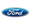•••###Bronco Rear Axles

Some available Rear Axle options for the bronco are:

Year:

## 1996 ford bronco Rear Axle

• ABS 3.08 ratio
• ABS 3.55 ratio
• w/o ABS 3.08 ratio
• w/o ABS 3.55 ratio

• Year:

## 1995 ford bronco Rear Axle

• w/o ABS 3.55 ratio
• w/o ABS 3.08 ratio
• ABS 3.08 ratio
• ABS 3.55 ratio

• Year:

## 1994 ford bronco Rear Axle

• w/o ABS 3.55 ratio
• ABS 3.08 ratio
• w/o ABS 3.08 ratio
• ABS 3.55 ratio

• Year:

## 1993 ford bronco Rear Axle

• ABS 3.08 ratio
• w/o ABS 3.55 ratio
• w/o ABS 3.08 ratio
• ABS 3.55 ratio

• Year:

## 1992 ford bronco Rear Axle

• ABS 3.55 ratio
• w/o ABS 3.55 ratio
• ABS 3.08 ratio
• ABS 4.10 ratio
• w/o ABS 3.08 ratio
• w/o ABS 4.10 ratio

• Year:

## 1991 ford bronco Rear Axle

• ABS 3.55 ratio
• ABS 4.10 ratio
• w/o ABS 3.08 ratio
• ABS 3.08 ratio
• w/o ABS 3.55 ratio
• w/o ABS 4.10 ratio

• Year:

## 1990 ford bronco Rear Axle

• w/o ABS 3.08 ratio
• ABS 3.55 ratio
• ABS 4.10 ratio
• w/o ABS 4.10 ratio
• w/o ABS 3.55 ratio
• ABS 3.08 ratio

• Year:

## 1989 ford bronco Rear Axle

• ABS 4.10 ratio
• ABS 3.55 ratio
• w/o ABS 3.08 ratio
• w/o ABS 4.10 ratio
• w/o ABS 3.55 ratio
• ABS 3.08 ratio

• Year:

## 1988 ford bronco Rear Axle

• w/o ABS 3.08 ratio
• ABS 3.55 ratio
• w/o ABS 3.55 ratio
• ABS 4.10 ratio
• w/o ABS 4.10 ratio
• ABS 3.08 ratio

• Year:

## 1987 ford bronco Rear Axle

• ABS 3.55 ratio
• w/o ABS 3.08 ratio
• w/o ABS 4.10 ratio
• ABS 3.08 ratio
• ABS 4.10 ratio
• w/o ABS 3.55 ratio

• Year:

## 1986 ford bronco Rear Axle

• non-removable carrier (8.8" ring gear) 3.08 ratio
• removable carrier (9" ring gear) 3.50 ratio
• removable carrier (9" ring gear) 4.11 ratio
• removable carrier (9" ring gear) 3.00 ratio
• non-removable carrier (8.8" ring gear) 3.55 ratio

• Year:

## 1985 ford bronco Rear Axle

• removable carrier (9" ring gear) 3.50 ratio
• non-removable carrier (8.8" ring gear) 3.08 ratio
• removable carrier (9" ring gear) 4.11 ratio
• removable carrier (9" ring gear) 3.00 ratio
• non-removable carrier (8.8" ring gear) 3.55 ratio

• Year:

## 1984 ford bronco Rear Axle

• non-removable carrier (8.8" ring gear) 3.55 ratio
• removable carrier (9" ring gear) 3.50 ratio
• removable carrier (9" ring gear) 4.11 ratio
• removable carrier (9" ring gear) 3.00 ratio
• non-removable carrier (8.8" ring gear) 3.08 ratio

• Year:

## 1983 ford bronco Rear Axle

• removable carrier (9" ring gear) 3.50 ratio
• removable carrier (9" ring gear) 3.00 ratio
• non-removable carrier (8.8" ring gear) 3.08 ratio
• removable carrier (9" ring gear) 4.11 ratio
• non-removable carrier (8.8" ring gear) 3.55 ratio

• Year:

## 1982 ford bronco Rear Axle

• (removable carrier, 9' ring gear) 3.50 ratio
• (removable carrier, 9' ring gear) 3.00 ratio
• (removable carrier, 9' ring gear) 4.11 ratio

• Year:

## 1981 ford bronco Rear Axle

• (removable carrier, 9' ring gear) 3.50 ratio
• (removable carrier, 9' ring gear) 3.00 ratio
• (removable carrier, 9' ring gear) 4.11 ratio

• Year:

## 1980 ford bronco Rear Axle

• (removable carrier, 9' ring gear) 3.50 ratio
• (removable carrier, 9' ring gear) 3.00 ratio
• (removable carrier, 9' ring gear) 4.11 ratio